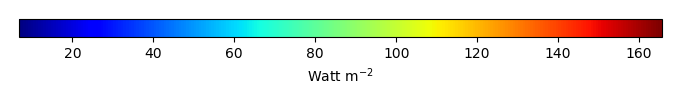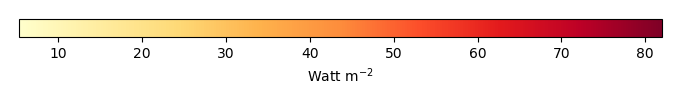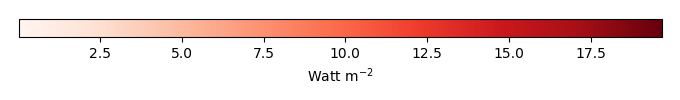# Mean State

Period Mean (original grids) [Watt m-2]
Model Period Mean (intersection) [Watt m-2]
Model Period Mean (complement) [Watt m-2]
Benchmark Period Mean (intersection) [Watt m-2]
Benchmark Period Mean (complement) [Watt m-2]
Bias [Watt m-2]
RMSE [Watt m-2]
Phase Shift [months]
Bias Score 
RMSE Score 
Seasonal Cycle Score 
Spatial Distribution Score 
Interannual Variability Score 
Overall Score 
Benchmark [-] 38.3
CLM4 [-] 36.8 36.5 39.6 15.5 -2.56 10.9 1.71 0.374 0.374 0.724 0.912 0.469 0.538
CLM4.5 [-] 36.4 36.1 39.6 15.5 -2.83 11.1 1.88 0.364 0.368 0.694 0.919 0.474 0.531
CLM5 [-] 36.3 36.0 39.6 15.5 -3.01 10.6 1.70 0.383 0.372 0.724 0.954 0.476 0.547
Period Mean (original grids) [Watt m-2]
Model Period Mean (intersection) [Watt m-2]
Model Period Mean (complement) [Watt m-2]
Benchmark Period Mean (intersection) [Watt m-2]
Benchmark Period Mean (complement) [Watt m-2]
Bias [Watt m-2]
RMSE [Watt m-2]
Phase Shift [months]
Bias Score 
RMSE Score 
Seasonal Cycle Score 
Spatial Distribution Score 
Interannual Variability Score 
Overall Score 
Benchmark [-] 52.3
CLM4 [-] 48.7 49.2 52.8 14.1 -3.75 12.9 1.25 0.484 0.380 0.842 0.985 0.547 0.603
CLM4.5 [-] 47.7 48.2 52.8 14.1 -4.69 13.3 1.18 0.452 0.381 0.847 0.987 0.547 0.599
CLM5 [-] 47.2 47.6 52.8 14.1 -5.21 13.3 1.35 0.443 0.392 0.834 0.988 0.533 0.597
Period Mean (original grids) [Watt m-2]
Model Period Mean (intersection) [Watt m-2]
Model Period Mean (complement) [Watt m-2]
Benchmark Period Mean (intersection) [Watt m-2]
Benchmark Period Mean (complement) [Watt m-2]
Bias [Watt m-2]
RMSE [Watt m-2]
Phase Shift [months]
Bias Score 
RMSE Score 
Seasonal Cycle Score 
Spatial Distribution Score 
Interannual Variability Score 
Overall Score 
Benchmark [-] 39.3
CLM4 [-] 37.4 37.4 40.4 16.6 -2.81 11.9 1.86 0.422 0.397 0.711 0.930 0.556 0.569
CLM4.5 [-] 36.8 36.8 40.4 16.6 -3.31 12.3 1.83 0.402 0.385 0.719 0.938 0.571 0.567
CLM5 [-] 36.9 36.9 40.4 16.6 -3.30 11.9 1.76 0.411 0.407 0.729 0.912 0.558 0.570
Period Mean (original grids) [Watt m-2]
Model Period Mean (intersection) [Watt m-2]
Model Period Mean (complement) [Watt m-2]
Benchmark Period Mean (intersection) [Watt m-2]
Benchmark Period Mean (complement) [Watt m-2]
Bias [Watt m-2]
RMSE [Watt m-2]
Phase Shift [months]
Bias Score 
RMSE Score 
Seasonal Cycle Score 
Spatial Distribution Score 
Interannual Variability Score 
Overall Score 
Benchmark [-] 31.6
CLM4 [-] 45.4 45.3 31.5 32.6 14.0 28.2 0.236 0.624 0.483 0.984 0.979 0.765 0.720
CLM4.5 [-] 39.3 39.2 31.5 32.6 9.07 22.5 0.241 0.730 0.554 0.984 0.903 0.716 0.740
CLM5 [-] 36.8 36.6 31.5 32.6 6.48 20.3 0.340 0.766 0.578 0.977 0.878 0.709 0.748
Period Mean (original grids) [Watt m-2]
Model Period Mean (intersection) [Watt m-2]
Model Period Mean (complement) [Watt m-2]
Benchmark Period Mean (intersection) [Watt m-2]
Benchmark Period Mean (complement) [Watt m-2]
Bias [Watt m-2]
RMSE [Watt m-2]
Phase Shift [months]
Bias Score 
RMSE Score 
Seasonal Cycle Score 
Spatial Distribution Score 
Interannual Variability Score 
Overall Score 
Benchmark [-] 23.5
CLM4 [-] 29.3 29.3 25.9 15.5 3.86 11.0 1.90 0.422 0.373 0.705 0.214 0.455 0.424
CLM4.5 [-] 29.3 29.3 25.9 15.5 3.86 11.0 1.89 0.420 0.375 0.704 0.227 0.449 0.425
CLM5 [-] 29.7 29.6 25.9 15.5 4.21 11.0 2.04 0.435 0.381 0.682 0.197 0.431 0.417
Period Mean (original grids) [Watt m-2]
Model Period Mean (intersection) [Watt m-2]
Model Period Mean (complement) [Watt m-2]
Benchmark Period Mean (intersection) [Watt m-2]
Benchmark Period Mean (complement) [Watt m-2]
Bias [Watt m-2]
RMSE [Watt m-2]
Phase Shift [months]
Bias Score 
RMSE Score 
Seasonal Cycle Score 
Spatial Distribution Score 
Interannual Variability Score 
Overall Score 
Benchmark [-] 21.4
CLM4 [-] 26.1 26.0 21.5 17.6 4.45 11.7 0.479 0.699 0.511 0.943 0.784 0.704 0.692
CLM4.5 [-] 28.0 27.9 21.5 17.6 6.30 13.8 0.515 0.646 0.471 0.946 0.697 0.681 0.652
CLM5 [-] 25.5 25.4 21.5 17.6 3.83 10.8 0.499 0.726 0.521 0.942 0.869 0.703 0.714
Period Mean (original grids) [Watt m-2]
Model Period Mean (intersection) [Watt m-2]
Model Period Mean (complement) [Watt m-2]
Benchmark Period Mean (intersection) [Watt m-2]
Benchmark Period Mean (complement) [Watt m-2]
Bias [Watt m-2]
RMSE [Watt m-2]
Phase Shift [months]
Bias Score 
RMSE Score 
Seasonal Cycle Score 
Spatial Distribution Score 
Interannual Variability Score 
Overall Score 
Benchmark [-] 30.0
CLM4 [-] 40.2 40.1 30.5 14.9 8.90 14.4 1.07 0.525 0.446 0.860 0.901 0.645 0.637
CLM4.5 [-] 40.9 40.9 30.5 14.9 9.57 14.9 1.07 0.500 0.440 0.858 0.902 0.648 0.631
CLM5 [-] 39.2 39.1 30.5 14.9 7.88 13.8 1.08 0.547 0.445 0.855 0.966 0.655 0.652
Period Mean (original grids) [Watt m-2]
Model Period Mean (intersection) [Watt m-2]
Model Period Mean (complement) [Watt m-2]
Benchmark Period Mean (intersection) [Watt m-2]
Benchmark Period Mean (complement) [Watt m-2]
Bias [Watt m-2]
RMSE [Watt m-2]
Phase Shift [months]
Bias Score 
RMSE Score 
Seasonal Cycle Score 
Spatial Distribution Score 
Interannual Variability Score 
Overall Score 
Benchmark [-] 29.5
CLM4 [-] 38.9 38.8 30.6 14.2 7.31 13.2 0.963 0.528 0.388 0.893 0.933 0.534 0.611
CLM4.5 [-] 38.2 38.2 30.6 14.2 6.70 13.1 0.893 0.512 0.392 0.897 0.937 0.510 0.607
CLM5 [-] 37.6 37.6 30.6 14.2 6.13 12.4 0.935 0.549 0.410 0.895 0.978 0.476 0.620
Period Mean (original grids) [Watt m-2]
Model Period Mean (intersection) [Watt m-2]
Model Period Mean (complement) [Watt m-2]
Benchmark Period Mean (intersection) [Watt m-2]
Benchmark Period Mean (complement) [Watt m-2]
Bias [Watt m-2]
RMSE [Watt m-2]
Phase Shift [months]
Bias Score 
RMSE Score 
Seasonal Cycle Score 
Spatial Distribution Score 
Interannual Variability Score 
Overall Score 
Benchmark [-] 33.0
CLM4 [-] 41.9 41.7 32.8 37.1 9.07 25.3 0.170 0.731 0.523 0.989 0.952 0.750 0.745
CLM4.5 [-] 40.1 39.9 32.8 37.1 7.69 24.6 0.295 0.747 0.544 0.980 0.980 0.677 0.745
CLM5 [-] 35.1 34.9 32.8 37.1 2.86 19.3 0.271 0.806 0.600 0.982 0.984 0.647 0.770
Period Mean (original grids) [Watt m-2]
Model Period Mean (intersection) [Watt m-2]
Model Period Mean (complement) [Watt m-2]
Benchmark Period Mean (intersection) [Watt m-2]
Benchmark Period Mean (complement) [Watt m-2]
Bias [Watt m-2]
RMSE [Watt m-2]
Phase Shift [months]
Bias Score 
RMSE Score 
Seasonal Cycle Score 
Spatial Distribution Score 
Interannual Variability Score 
Overall Score 
Benchmark [-] 35.1
CLM4 [-] 41.9 41.9 36.7 14.1 4.04 10.6 0.638 0.588 0.542 0.952 0.935 0.596 0.693
CLM4.5 [-] 41.8 41.8 36.7 14.1 3.97 10.5 0.650 0.594 0.540 0.950 0.945 0.600 0.695
CLM5 [-] 40.8 40.8 36.7 14.1 3.07 10.8 0.654 0.579 0.530 0.948 0.900 0.637 0.688
Period Mean (original grids) [Watt m-2]
Model Period Mean (intersection) [Watt m-2]
Model Period Mean (complement) [Watt m-2]
Benchmark Period Mean (intersection) [Watt m-2]
Benchmark Period Mean (complement) [Watt m-2]
Bias [Watt m-2]
RMSE [Watt m-2]
Phase Shift [months]
Bias Score 
RMSE Score 
Seasonal Cycle Score 
Spatial Distribution Score 
Interannual Variability Score 
Overall Score 
Benchmark [-] 19.8
CLM4 [-] 27.8 27.8 20.7 10.0 6.44 13.0 0.866 0.619 0.503 0.909 0.926 0.672 0.688
CLM4.5 [-] 26.9 26.9 20.7 10.0 5.62 11.8 1.08 0.622 0.505 0.871 0.974 0.688 0.694
CLM5 [-] 26.8 26.9 20.7 10.0 5.61 11.4 1.02 0.622 0.523 0.888 0.973 0.675 0.701
Period Mean (original grids) [Watt m-2]
Model Period Mean (intersection) [Watt m-2]
Model Period Mean (complement) [Watt m-2]
Benchmark Period Mean (intersection) [Watt m-2]
Benchmark Period Mean (complement) [Watt m-2]
Bias [Watt m-2]
RMSE [Watt m-2]
Phase Shift [months]
Bias Score 
RMSE Score 
Seasonal Cycle Score 
Spatial Distribution Score 
Interannual Variability Score 
Overall Score 
Benchmark [-] 23.6
CLM4 [-] 49.0 49.0 43.1 14.1 4.96 17.5 0.872 0.575 0.475 0.889 0.871 0.565 0.642
CLM4.5 [-] 48.4 48.4 43.1 14.1 4.45 17.0 0.908 0.568 0.476 0.883 0.853 0.563 0.636
CLM5 [-] 47.6 47.6 43.1 14.1 3.75 16.6 0.898 0.586 0.485 0.886 0.880 0.562 0.647
Period Mean (original grids) [Watt m-2]
Model Period Mean (intersection) [Watt m-2]
Model Period Mean (complement) [Watt m-2]
Benchmark Period Mean (intersection) [Watt m-2]
Benchmark Period Mean (complement) [Watt m-2]
Bias [Watt m-2]
RMSE [Watt m-2]
Phase Shift [months]
Bias Score 
RMSE Score 
Seasonal Cycle Score 
Spatial Distribution Score 
Interannual Variability Score 
Overall Score 
Benchmark [-] 64.3
CLM4 [-] 83.3 83.2 66.2 16.5 15.0 21.9 0.619 0.505 0.556 0.942 0.985 0.510 0.676
CLM4.5 [-] 82.7 82.5 66.2 16.5 14.3 21.5 0.633 0.513 0.557 0.940 0.985 0.514 0.678
CLM5 [-] 81.5 81.3 66.2 16.5 13.1 21.0 0.697 0.529 0.548 0.934 0.983 0.535 0.680
Period Mean (original grids) [Watt m-2]
Model Period Mean (intersection) [Watt m-2]
Model Period Mean (complement) [Watt m-2]
Benchmark Period Mean (intersection) [Watt m-2]
Benchmark Period Mean (complement) [Watt m-2]
Bias [Watt m-2]
RMSE [Watt m-2]
Phase Shift [months]
Bias Score 
RMSE Score 
Seasonal Cycle Score 
Spatial Distribution Score 
Interannual Variability Score 
Overall Score 
Benchmark [-] 34.3
CLM4 [-] 44.8 44.8 34.2 35.0 11.8 26.3 0.284 0.675 0.534 0.979 0.963 0.735 0.737
CLM4.5 [-] 43.2 43.1 34.2 35.0 10.5 24.7 0.336 0.700 0.560 0.975 0.943 0.681 0.736
CLM5 [-] 42.0 41.9 34.2 35.0 9.42 24.2 0.362 0.711 0.561 0.973 0.945 0.675 0.737
Period Mean (original grids) [Watt m-2]
Model Period Mean (intersection) [Watt m-2]
Model Period Mean (complement) [Watt m-2]
Benchmark Period Mean (intersection) [Watt m-2]
Benchmark Period Mean (complement) [Watt m-2]
Bias [Watt m-2]
RMSE [Watt m-2]
Phase Shift [months]
Bias Score 
RMSE Score 
Seasonal Cycle Score 
Spatial Distribution Score 
Interannual Variability Score 
Overall Score 
Benchmark [-] 24.8
CLM4 [-] 33.9 33.8 27.0 14.3 6.23 10.9 0.858 0.516 0.439 0.909 0.900 0.552 0.626
CLM4.5 [-] 34.3 34.2 27.0 14.3 6.90 11.3 0.888 0.486 0.435 0.906 0.850 0.559 0.612
CLM5 [-] 34.1 34.1 27.0 14.3 6.78 11.3 0.907 0.478 0.447 0.901 0.791 0.543 0.601
Period Mean (original grids) [Watt m-2]
Model Period Mean (intersection) [Watt m-2]
Model Period Mean (complement) [Watt m-2]
Benchmark Period Mean (intersection) [Watt m-2]
Benchmark Period Mean (complement) [Watt m-2]
Bias [Watt m-2]
RMSE [Watt m-2]
Phase Shift [months]
Bias Score 
RMSE Score 
Seasonal Cycle Score 
Spatial Distribution Score 
Interannual Variability Score 
Overall Score 
Benchmark [-] 20.7
CLM4 [-] 29.1 28.9 21.1 12.1 7.45 11.0 0.779 0.500 0.465 0.902 0.981 0.633 0.658
CLM4.5 [-] 30.0 29.8 21.1 12.1 8.34 11.9 1.05 0.456 0.441 0.853 0.948 0.674 0.636
CLM5 [-] 30.7 30.6 21.1 12.1 9.05 12.2 1.02 0.422 0.453 0.855 0.933 0.654 0.628
Period Mean (original grids) [Watt m-2]
Model Period Mean (intersection) [Watt m-2]
Model Period Mean (complement) [Watt m-2]
Benchmark Period Mean (intersection) [Watt m-2]
Benchmark Period Mean (complement) [Watt m-2]
Bias [Watt m-2]
RMSE [Watt m-2]
Phase Shift [months]
Bias Score 
RMSE Score 
Seasonal Cycle Score 
Spatial Distribution Score 
Interannual Variability Score 
Overall Score 
Benchmark [-] 20.3
CLM4 [-] 26.9 27.1 20.6 10.6 6.40 12.9 0.692 0.605 0.494 0.919 0.701 0.637 0.642
CLM4.5 [-] 26.0 26.1 20.6 10.6 5.46 12.0 0.694 0.619 0.500 0.919 0.804 0.621 0.661
CLM5 [-] 26.2 26.3 20.6 10.6 5.71 11.9 0.662 0.607 0.512 0.916 0.830 0.644 0.670
Period Mean (original grids) [Watt m-2]
Model Period Mean (intersection) [Watt m-2]
Model Period Mean (complement) [Watt m-2]
Benchmark Period Mean (intersection) [Watt m-2]
Benchmark Period Mean (complement) [Watt m-2]
Bias [Watt m-2]
RMSE [Watt m-2]
Phase Shift [months]
Bias Score 
RMSE Score 
Seasonal Cycle Score 
Spatial Distribution Score 
Interannual Variability Score 
Overall Score 
Benchmark [-] 30.8
CLM4 [-] 37.6 37.6 31.1 10.8 5.98 11.7 0.722 0.576 0.487 0.901 0.944 0.648 0.674
CLM4.5 [-] 38.7 38.7 31.1 10.8 7.17 12.3 1.01 0.529 0.495 0.846 0.922 0.697 0.664
CLM5 [-] 36.0 36.0 31.1 10.8 4.52 10.7 0.919 0.626 0.497 0.874 0.988 0.723 0.701
Period Mean (original grids) [Watt m-2]
Model Period Mean (intersection) [Watt m-2]
Model Period Mean (complement) [Watt m-2]
Benchmark Period Mean (intersection) [Watt m-2]
Benchmark Period Mean (complement) [Watt m-2]
Bias [Watt m-2]
RMSE [Watt m-2]
Phase Shift [months]
Bias Score 
RMSE Score 
Seasonal Cycle Score 
Spatial Distribution Score 
Interannual Variability Score 
Overall Score 
Benchmark [-] 26.8
CLM4 [-] 28.8 28.8 27.1 15.2 1.72 8.45 1.72 0.682 0.370 0.750 0.703 0.425 0.550
CLM4.5 [-] 28.6 28.6 27.1 15.2 1.66 8.48 1.72 0.686 0.368 0.752 0.740 0.429 0.557
CLM5 [-] 28.8 28.8 27.1 15.2 1.84 8.42 1.63 0.686 0.372 0.766 0.710 0.417 0.554
Period Mean (original grids) [Watt m-2]
Model Period Mean (intersection) [Watt m-2]
Model Period Mean (complement) [Watt m-2]
Benchmark Period Mean (intersection) [Watt m-2]
Benchmark Period Mean (complement) [Watt m-2]
Bias [Watt m-2]
RMSE [Watt m-2]
Phase Shift [months]
Bias Score 
RMSE Score 
Seasonal Cycle Score 
Spatial Distribution Score 
Interannual Variability Score 
Overall Score 
Benchmark [-] 29.5
CLM4 [-] 31.8 31.8 29.9 14.5 1.95 9.35 1.39 0.705 0.439 0.819 0.763 0.520 0.614
CLM4.5 [-] 31.9 31.8 29.9 14.5 1.98 9.36 1.48 0.718 0.435 0.805 0.749 0.529 0.612
CLM5 [-] 32.7 32.6 29.9 14.5 2.75 9.25 1.19 0.693 0.452 0.843 0.687 0.495 0.604
Period Mean (original grids) [Watt m-2]
Model Period Mean (intersection) [Watt m-2]
Model Period Mean (complement) [Watt m-2]
Benchmark Period Mean (intersection) [Watt m-2]
Benchmark Period Mean (complement) [Watt m-2]
Bias [Watt m-2]
RMSE [Watt m-2]
Phase Shift [months]
Bias Score 
RMSE Score 
Seasonal Cycle Score 
Spatial Distribution Score 
Interannual Variability Score 
Overall Score 
Benchmark [-] 28.0
CLM4 [-] 35.3 35.4 29.7 12.6 5.35 14.5 0.785 0.488 0.467 0.909 0.865 0.515 0.618
CLM4.5 [-] 34.8 34.9 29.7 12.6 4.85 14.1 0.814 0.486 0.469 0.901 0.825 0.536 0.614
CLM5 [-] 33.5 33.5 29.7 12.6 3.52 13.5 0.829 0.504 0.473 0.908 0.753 0.545 0.609

# Temporally integrated period mean

BENCHMARK MEANMODEL MEANBIASBIAS SCORERMSERMSE SCOREBENCHMARK INTERANNUAL VARIABILITYMODEL INTERANNUAL VARIABILITYINTERANNUAL VARIABILITY SCOREBENCHMARK MAX MONTHMODEL MAX MONTHDIFFERENCE IN MAX MONTHSEASONAL CYCLE SCORESPATIAL TAYLOR DIAGRAMMODEL COLORS# Spatially integrated regional mean

MODEL COLORSREGIONAL MEANANNUAL CYCLEMONTHLY ANOMALYANNUAL CYCLE# All Models

BenchmarkCLM4CLM4.5CLM5# Data Information

creation_date: Mon Jun 30 23:28:16 PDT 2014

source_file: This product is generated from monthly 1 degree GEWEX SRB Radiation observations